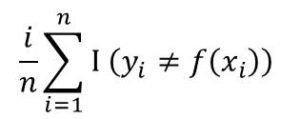# Machine Learning 101 – Introduction to Classification

In subsequent lectures, we have  discussed regression problems. Now we would apply the same analysis to classification but with little adjustment.

In case of classification, we also have a dataset made up of x and y. However, values of y is now discreet or categorical. It’s not continuous as in the case of regression.

Another difference about the model accuracy. In regression, the model accuracy was improved by reducing the Mean-Squared-Error(MSE). But here in classification, we would be interested in the error rate.

The error rate computes the proportion of misclassifications that is made.  It is given by the formula:Here f(xi) is the predicted class label for the ith observation using f.

I(yi ≠ f(xi) is an indicator random variable that counts is equal to 1 when yi ≠ f(xi) and equals zero when yi = f(xi).

If I = 0 for the ith observation, then xi was classified correctly. Otherwise, I = 1 meaning that xi was misclassified. Therefore the formula gives us the fraction of incorrect classifications.

When we apply our model to the training data set, then we could compute the training error rate. However, we are interested in in the test error rate. That is the error rate when the classifier is applied to a test data set.

The test error rate for the for a set of test data of the form (x0, y0) is given by:

Ave(I(y0 ≠  f(x0))

where y0 is the predicted class label using our classifier.

As before, the objective is the minimize the test error rates.

#### Example of Classification Problem

Let’s take a medical diagnosis example. Here, an X-ray of the patient is taken and using this image, the physicians need to determine if the patient has cancer or not. So the output class are:

• C1 denoting the presence of cancer
• C2 denoting the absence of cancer.

So the input can be the X-rag image which could be considered as input vector x (set of pixel intensities of the image). The output or target variable is t which is denoted as C1 and C2.

So the question is:

What is the probability that an X-ray image has certain set of pixel intensities and the patient have cancer?

or

What is the probability that an X-ray image has certain set of pixel intensities and the  patient does not have cancer?

There is a two step approach to this problem:

The first is inference. That is to determine the joint probability distribution p(x, Cx). This is same as p(x, t)

The second is decision. Given this probability, then make a decision on which action to take

This is what forms the basis of Decision Theory. This is a brief video I made on decision theory.

This can be solved using Bayes’ Classifier.  I recommend you review Bayes’ theorem since the next lectures in classification assumes you have a knowledge of Bayes’ Theorem.

Also review Probability Theory generally as you would need it to understand Decision Theory.

Quiz

What are the three fundamental theories of machine learning?

Leave your answer in the comment box below#### kindsonthegenius

Kindson Munonye is currently completing his doctoral program in Software Engineering in Budapest University of Technology and Economics

View all posts by kindsonthegenius →